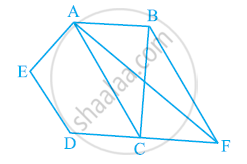# In the given figure, ABCDE is a pentagon. A line through B parallel to AC meets DC produced at F. Show that - Mathematics

In the given figure, ABCDE is a pentagon. A line through B parallel to AC meets DC produced at F. Show that

(i) ar (ACB) = ar (ACF)

(ii) ar (AEDF) = ar (ABCDE)#### Solution

(i) ΔACB and ΔACF lie on the same base AC and are between

The same parallels AC and BF.

∴ Area (ΔACB) = Area (ΔACF)

(ii) It can be observed that

Area (ΔACB) = Area (ΔACF)

⇒ Area (ΔACB) + Area (ACDE) = Area (ACF) + Area (ACDE)

⇒ Area (ABCDE) = Area (AEDF)

Concept: Corollary: Triangles on the same base and between the same parallels are equal in area.
Is there an error in this question or solution?
Chapter 9: Areas of Parallelograms and Triangles - Exercise 9.3 [Page 163]

#### APPEARS IN

NCERT Class 9 Maths
Chapter 9 Areas of Parallelograms and Triangles
Exercise 9.3 | Q 11 | Page 163

Share